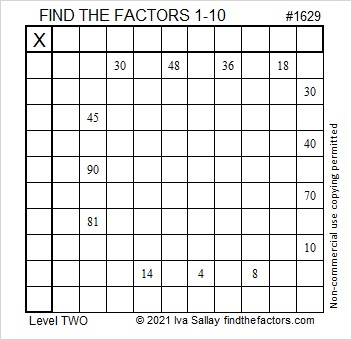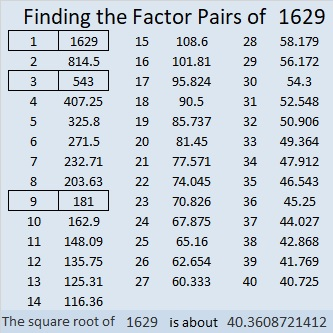# 1629 and Level 2

Contents

### Today’s Puzzle:

Write the numbers from 1 to 10 in both the first column and the top row so that those numbers and the given clues work to make a multiplication table.### Factors of 1629:

1 + 6 + 2 = 9, so 1929 is divisible by both 3 and 9. (It’s only necessary to add the non-nine numbers together to check those two divisibility rules.)

• 1629 is a composite number.
• Prime factorization: 1629 = 3 × 3 × 181, which can be written 1629 = 3² × 181.
• 1629 has at least one exponent greater than 1 in its prime factorization so √1629 can be simplified. Taking the factor pair from the factor pair table below with the largest square number factor, we get √1629 = (√9)(√181) = 3√181.
• The exponents in the prime factorization are 2 and 1. Adding one to each exponent and multiplying we get (2 + 1)(1 + 1) = 3 × 2 = 6. Therefore 1629 has exactly 6 factors.
• The factors of 1629 are outlined with their factor pair partners in the graphic below.### More about the Number 1629:

1629 is the sum of two squares:
30² + 27² = 1629.

1629 is the hypotenuse of a Pythagorean triple:
171-1620-1629, calculated from 30² – 27², 2(30)(27), 30² + 27².
It is also 9 times (19-180-181).

This site uses Akismet to reduce spam. Learn how your comment data is processed.